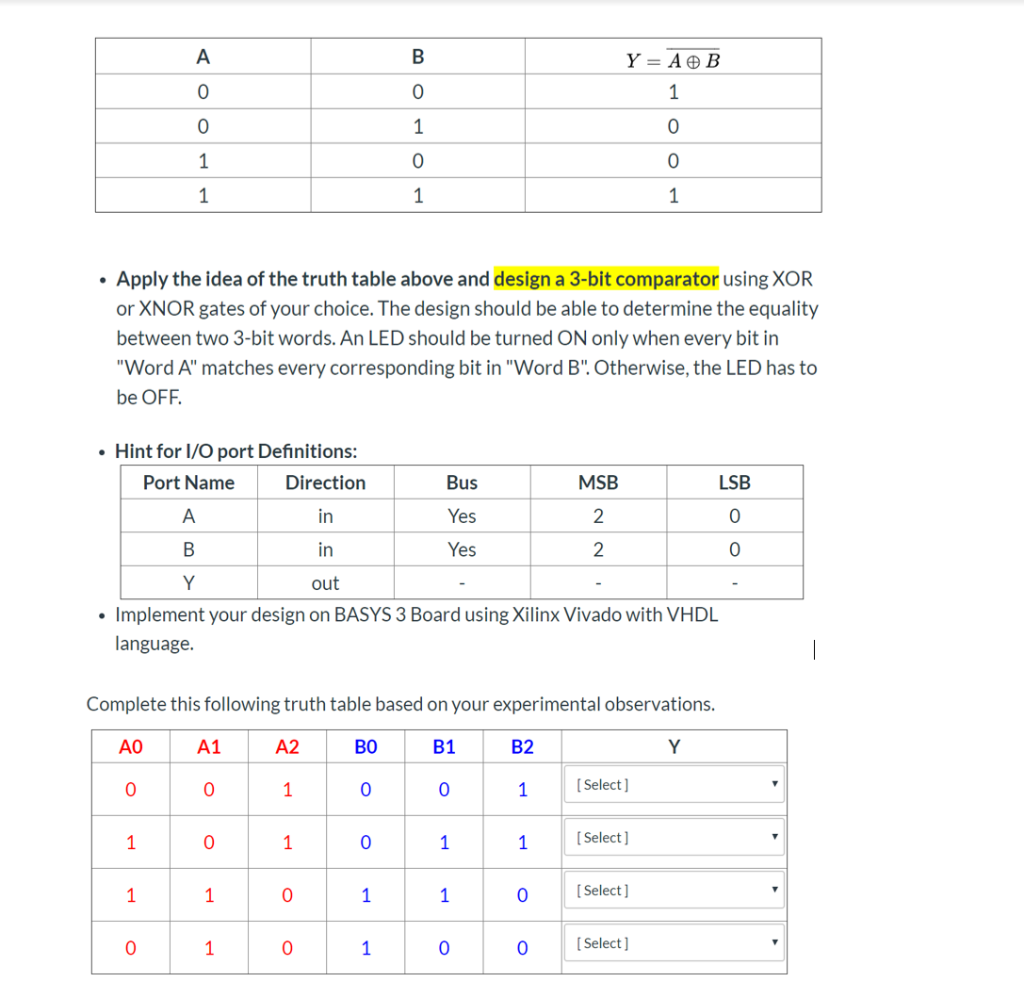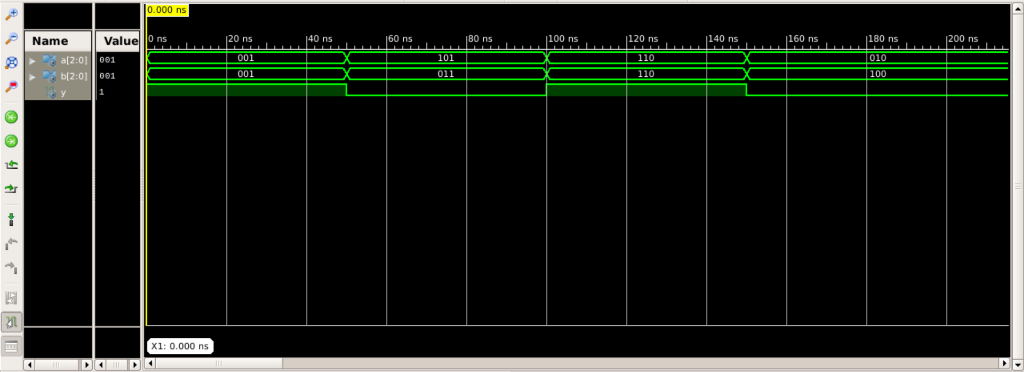# A Y = A ® B 1 1 1 1 1 Apply the idea of the truth table above and design a 3-bit comparator using XOR or XNOR gates of...A Y = A ® B 1 1 1 1 1 Apply the idea of the truth table above and design a 3-bit comparator using XOR or XNOR gates of your choice. The design should be able to determine the equality between two 3-bit words. An LED should be turned ON only when every bit in "Word A" matches every corresponding bit in "Word B". Otherwise, the LED has to be OFF Hint for I/O port Definitions: Port Name Direction Bus MSB LSB A in Yes 2 Yes in 2 Y out Implement your design on BASYS 3 Board using Xilinx Vivado with VHDL language Complete this following truth table based on your experimental observations. AO A1 A2 BO B1 B2 Y [ Select ] 1 1 Select 1 1 0 1 1 [Select] 1 1 0 1 1 [Select] 1 0 C

VHDL Code for the 3 bit equality comparator is below:

-- In the 1 bit exnor gate, when the two inputs A abd B are equal then we get the Y = '1';
-- so we will use this feature of exnor gate to form a 3 bit comparator

library ieee;
use ieee.std_logic_1164.all;
use ieee.std_logic_arith.all;

entity comparator is
port(
A : in std_logic_vector (2 downto 0);
B : in std_logic_vector (2 downto 0);
Y : out std_logic
);
end comparator;

architecture behave of comparator is
signal eq0, eq1, eq2 : std_logic;
begin

eq2 <= not (A(2) xor B(2));
eq1 <= not (A(1) xor B(1));
eq0 <= not (A(0) xor B(0));
-- all of the bits are equal then only Y = '1' else not
Y <= eq0 and eq1 and eq2;

end behave;

VHDL Testbench code for the above design code is:

library ieee;
use ieee.std_logic_1164.all;

entity comparator_tb is
end comparator_tb;

architecture behavior of comparator_tb is

component comparator
port(
A : in std_logic_vector(2 downto 0);
B : in std_logic_vector(2 downto 0);
Y : out std_logic
);
end component;

--Inputs
signal A : std_logic_vector(2 downto 0) := (others => '0');
signal B : std_logic_vector(2 downto 0) := (others => '0');

--Outputs
signal Y : std_logic;

begin

-- Instantiate the Unit Under Test (UUT)
uut: comparator port map (
A => A,
B => B,
Y => Y
);

-- Stimulus process
stim_proc: process
begin
A <= "001"; B <= "001"; wait for 50 ns;
A <= "101"; B <= "011"; wait for 50 ns;
A <= "110"; B <= "110"; wait for 50 ns;
A <= "010"; B <= "100"; wait for 50 ns;
wait;
end process;

end;

Wave form for the output :-##### Add Answer of: A Y = A ® B 1 1 1 1 1 Apply the idea of the truth table above and design a 3-bit comparator using XOR or XNOR gates of...
More Homework Help Questions Additional questions in this topic.